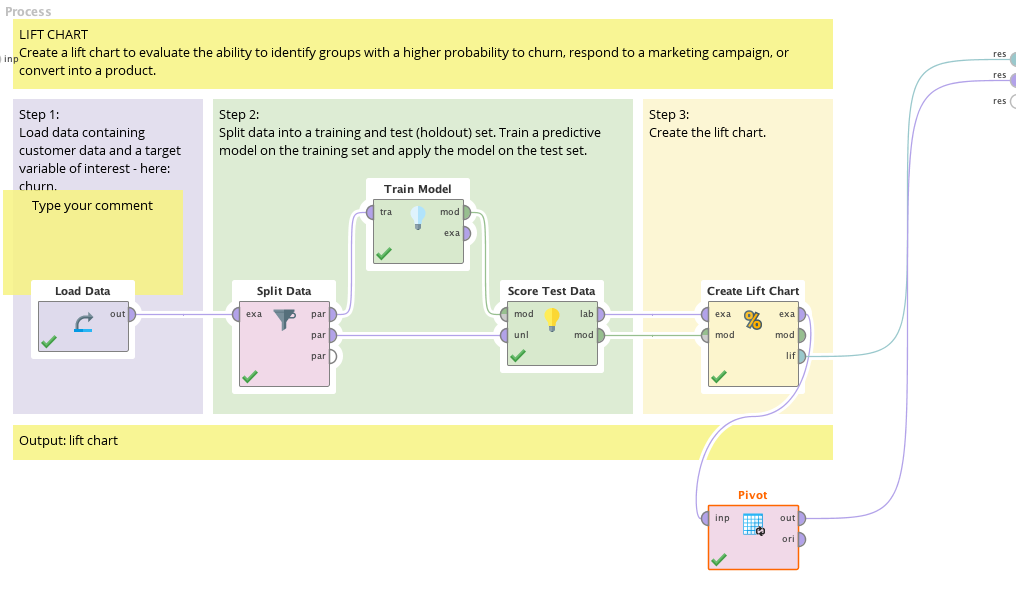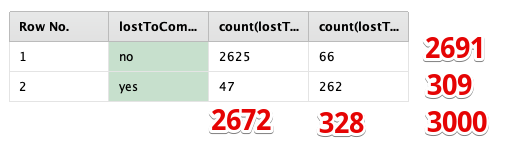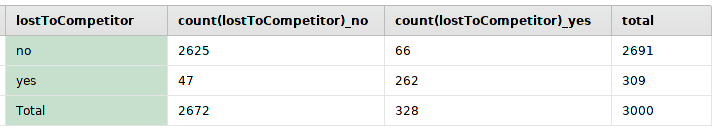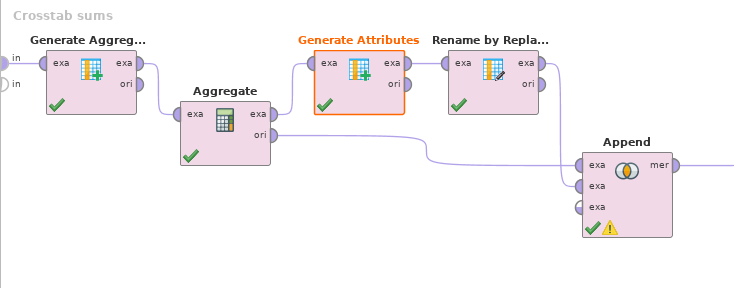# Simple Crosstab with totals

Member, University Professor Posts: 68Maven
edited September 2019 in Help
I have seen other questions posted here and via Google Searches, but perhaps the solution was for prior RM versions where operators are either no longer available, or are now required paid licenses via 3rd party tools.

My thought was to simply Pivot the data, which works as expected, but the ability to add a total is lacking.  In my case, it's just a simple 2x2, which I can do with pivot, but the table is lacking totals.

Could I add them up, sure?  But that feels clunky given how powerful, and intuitive, the tool is to use.

Is is not possible without paying for a license via an extension found in the Marketplace?  I am really going to enjoy using this as a teaching tool for my class, but it feels like I must be missing something obvious when generating simple 2x2 tables, with totals, is basically EDA 101.

Thanks,

Brock
Tagged:

• Administrator, Moderator, Employee, RapidMiner Certified Analyst, RapidMiner Certified Expert, University Professor Posts: 2,221RM Data Scientist
edited September 2019

can you give me an example (for example in excel) how this might look like? Let me see, maybe I find the time to build it.

Cheers,
Martin

- Head of Data Science Services at RapidMiner -
Dortmund, Germany
• Member, University Professor Posts: 68Maven
Thanks for the quick reply.  I was just playing around with the built in tutorial for lift charts, and modified the output to include pivot.  The attached screenshots show the process and the expected output.   From the example, I am just crossing the label with the predicted value into a 2x2 matrix.

And to your other question, those examples are definitely more complicated than I am looking for out of the box.

Cheers,

Brock• 12Administrator, Moderator, Employee, RapidMiner Certified Analyst, Community Manager, Member, University Professor, PM Moderator Posts: 2,717Community Manager
hi @btibertb yeah that seems like one of those things where simply 'no one has ever asked before'. It can probably be done very simply with a few lines of code, or perhaps you can create a BuildingBlock for people to use?

Scott
• Administrator, Moderator, Employee, RapidMiner Certified Analyst, RapidMiner Certified Expert, University Professor Posts: 2,221RM Data Scientist
Hey @btibert ,
i've got 20 minutes to write a prototype in java for it. Are you interested polishing it and creating your own extension? The code for the operator is this:
`package com.rapidminer.extension.operator.blending;import java.util.ArrayList;import java.util.Arrays;import java.util.List;import com.rapidminer.example.Attribute;import com.rapidminer.example.Example;import com.rapidminer.example.ExampleSet;import com.rapidminer.example.table.AttributeFactory;import com.rapidminer.example.utils.ExampleSetBuilder;import com.rapidminer.example.utils.ExampleSets;import com.rapidminer.operator.Operator;import com.rapidminer.operator.OperatorDescription;import com.rapidminer.operator.UserError;import com.rapidminer.operator.ports.InputPort;import com.rapidminer.operator.ports.OutputPort;import com.rapidminer.parameter.ParameterType;import com.rapidminer.parameter.ParameterTypeAttribute;import com.rapidminer.parameter.ParameterTypeBoolean;import com.rapidminer.parameter.ParameterTypeCategory;import com.rapidminer.parameter.ParameterTypeDouble;import com.rapidminer.parameter.ParameterTypeInt;import com.rapidminer.parameter.conditions.EqualStringCondition;import com.rapidminer.tools.Ontology;public class CrossTable extends Operator {   InputPort exaInput = getInputPorts().createPort("exa");   OutputPort crossOutput = getOutputPorts().createPort("cro");   OutputPort crossOut = getOutputPorts().createPassThroughPort("ori");   public final static String PARAMETER_X_ATTRIBTUE = "x_attribute";   public final static String PARAMETER_Y_ATTRIBTUE = "y_attribute";   public CrossTable(OperatorDescription description) {      super(description);   }   public void doWork() throws UserError {      ExampleSet exampleSet = exaInput.getData(ExampleSet.class);      Attribute x = exampleSet.getAttributes().get(getParameterAsString(PARAMETER_X_ATTRIBTUE));      Attribute y = exampleSet.getAttributes().get(getParameterAsString(PARAMETER_Y_ATTRIBTUE));      Integer xSize = x.getMapping().getValues().size();      Integer ySize = y.getMapping().getValues().size();      double[][] values = new double[xSize + 1][ySize + 2];      // Initialize with 0s      for (int xCounter = 0; xCounter < xSize + 1; ++xCounter) {         for (int yCounter = 1; yCounter < ySize + 2; ++yCounter) {            values[xCounter][yCounter] = 0;         }      }      // Add the names      Attribute nameAttribute = AttributeFactory.createAttribute("name",Ontology.POLYNOMINAL);      for (int xCounter = 0; xCounter < xSize; ++xCounter) {         values[xCounter] = nameAttribute.getMapping().mapString(x.getMapping().getValues().get(xCounter));      }      values[xSize] =  nameAttribute.getMapping().mapString("sum");      // sum!for (Example exa : exampleSet) {         int xIndex = x.getMapping().getValues().indexOf(exa.getNominalValue(x));         int yIndex = y.getMapping().getValues().indexOf(exa.getNominalValue(y));         values[xIndex][yIndex+1] += 1;      }      for (int xCounter = 0; xCounter < xSize; ++xCounter) {         double ySum = 0;         for (int yCounter = 0; yCounter < ySize; ++yCounter) {            ySum += values[xCounter][yCounter+1];         }         values[xCounter][ySize+1] = ySum;      }      for (int yCounter = 0; yCounter < ySize; ++yCounter) {         double xSum = 0;         for (int xCounter = 0; xCounter < xSize; ++xCounter) {            xSum += values[xCounter][yCounter+1];         }         values[xSize][yCounter+1] = xSum;      }      values[xSize][ySize+1] = exampleSet.size();      List<Attribute> listOfAttributes = new ArrayList<Attribute>();      listOfAttributes.add(nameAttribute);      for (String value : y.getMapping().getValues()) {         listOfAttributes.add(AttributeFactory.createAttribute("count(" + value + ")", Ontology.REAL));      }      listOfAttributes.add(AttributeFactory.createAttribute("sum", Ontology.REAL));      ExampleSetBuilder builder = ExampleSets.from(listOfAttributes);      for(double[] row : values){         builder.addRow(row);      }      crossOutput.deliver(builder.build());   }@Override   public List<ParameterType> getParameterTypes() {      List<ParameterType> types = new ArrayList<>();      types.add(new ParameterTypeAttribute(PARAMETER_X_ATTRIBTUE,"Attribute to construct the horizontal axis.",exaInput));      types.add(new ParameterTypeAttribute(PARAMETER_Y_ATTRIBTUE,"Attribute to construct the vertical axis.",exaInput));      return types;   }}`

Best,
Martin

- Head of Data Science Services at RapidMiner -
Dortmund, Germany
• Administrator, Moderator, Employee, RapidMiner Certified Analyst, RapidMiner Certified Expert Posts: 346Unicorn
Hi,

you can do it in RapidMiner.It needs a few steps:Unfortunately, it's not a good building block (yet) because the first (grouping) attribute name is hard coded.

Generate Aggregation creates the "total" attribute with the sum of all numeric columns (attribute filter type=value_type, value type=numeric).

Aggregate creates the sums with "use default aggregation", attribute filter type ... numeric, and default aggregation function = sum.

Then we add the text "Total" with the attribute name from the un-aggregated example set and rename the aggregated attributes from "sum(whatever)" to just "whatever" using Rename by Replacing (replace what: sum.(.+).\$ ; replace by: \$1).

Maybe we could extract the attribute name from the incoming example set, save it as a macro and reuse that in the Generate Attributes step. Then it would be completely generic and a possible building block. But that's an exercise for the reader ;-)

Regards,

Balázs
• Member, University Professor Posts: 68Maven
Wow, this is absolutely fantastic, thank you everyone.  Let me digest this (I am prepping for class tonight) but I am very encouraged by the ideas and feedback above.  Excellent stuff!
• Member, University Professor Posts: 68Maven
mschmitz  absolutely interested in learning how to do that, I am not quite there yet.
• Member, University Professor Posts: 68Maven
@BalazsBarany how would I go about adding that to my local install?  Thanks for your help on this!
• Member, University Professor Posts: 68Maven
NVM, I figured that part out.  I was looking for a way to "install" via the interface, but realized I can drop the file inside the install folder.  Thanks again.
• RapidMiner Certified Analyst, Member Posts: 79Unicorn
@btibert
Maybe my solution is not the easiest but it works:
```<?xml version="1.0" encoding="UTF-8"?><process version="9.3.001">
<context>
<input/>
<output/>
<macros/>
</context>
<operator activated="true" class="process" compatibility="9.3.001" expanded="true" name="Process">
<parameter key="logverbosity" value="init"/>
<parameter key="random_seed" value="2001"/>
<parameter key="send_mail" value="never"/>
<parameter key="process_duration_for_mail" value="30"/>
<parameter key="encoding" value="SYSTEM"/>
<process expanded="true">
<operator activated="true" class="generate_nominal_data" compatibility="9.3.001" expanded="true" height="68" name="Generate Nominal Data" width="90" x="45" y="34">
<parameter key="number_examples" value="100"/>
<parameter key="number_of_attributes" value="1"/>
<parameter key="number_of_values" value="5"/>
<parameter key="use_local_random_seed" value="false"/>
<parameter key="local_random_seed" value="1992"/>
</operator>
<operator activated="true" class="blending:pivot" compatibility="9.3.001" expanded="true" height="82" name="Pivot" width="90" x="179" y="34">
<parameter key="group_by_attributes" value="att1"/>
<parameter key="column_grouping_attribute" value="label"/>
<list key="aggregation_attributes">
<parameter key="label" value="count"/>
</list>
<parameter key="use_default_aggregation" value="false"/>
<parameter key="default_aggregation_function" value="first"/>
</operator>
<operator activated="true" class="rename_by_replacing" compatibility="9.3.001" expanded="true" height="82" name="Rename by Replacing" width="90" x="313" y="34">
<parameter key="attribute_filter_type" value="single"/>
<parameter key="attribute" value="att1"/>
<parameter key="attributes" value=""/>
<parameter key="use_except_expression" value="false"/>
<parameter key="value_type" value="attribute_value"/>
<parameter key="use_value_type_exception" value="false"/>
<parameter key="except_value_type" value="time"/>
<parameter key="block_type" value="attribute_block"/>
<parameter key="use_block_type_exception" value="false"/>
<parameter key="except_block_type" value="value_matrix_row_start"/>
<parameter key="invert_selection" value="true"/>
<parameter key="include_special_attributes" value="false"/>
<parameter key="replace_what" value=".*_"/>
</operator>
<operator activated="true" class="replace_missing_values" compatibility="9.3.001" expanded="true" height="103" name="Replace Missing Values" width="90" x="514" y="34">
<parameter key="return_preprocessing_model" value="false"/>
<parameter key="create_view" value="false"/>
<parameter key="attribute_filter_type" value="all"/>
<parameter key="attribute" value=""/>
<parameter key="attributes" value=""/>
<parameter key="use_except_expression" value="false"/>
<parameter key="value_type" value="attribute_value"/>
<parameter key="use_value_type_exception" value="false"/>
<parameter key="except_value_type" value="time"/>
<parameter key="block_type" value="attribute_block"/>
<parameter key="use_block_type_exception" value="false"/>
<parameter key="except_block_type" value="value_matrix_row_start"/>
<parameter key="invert_selection" value="false"/>
<parameter key="include_special_attributes" value="false"/>
<parameter key="default" value="zero"/>
<list key="columns"/>
</operator>
<operator activated="true" class="generate_aggregation" compatibility="9.3.001" expanded="true" height="82" name="Generate Aggregation" width="90" x="648" y="34">
<parameter key="attribute_name" value="Total_Column"/>
<parameter key="attribute_filter_type" value="single"/>
<parameter key="attribute" value="att1"/>
<parameter key="attributes" value=""/>
<parameter key="use_except_expression" value="false"/>
<parameter key="value_type" value="attribute_value"/>
<parameter key="use_value_type_exception" value="false"/>
<parameter key="except_value_type" value="time"/>
<parameter key="block_type" value="attribute_block"/>
<parameter key="use_block_type_exception" value="false"/>
<parameter key="except_block_type" value="value_matrix_row_start"/>
<parameter key="invert_selection" value="true"/>
<parameter key="include_special_attributes" value="false"/>
<parameter key="aggregation_function" value="sum"/>
<parameter key="concatenation_separator" value="|"/>
<parameter key="keep_all" value="true"/>
<parameter key="ignore_missings" value="true"/>
<parameter key="ignore_missing_attributes" value="false"/>
</operator>
<operator activated="true" class="transpose" compatibility="9.3.001" expanded="true" height="82" name="Transpose" width="90" x="782" y="34"/>
<operator activated="true" class="rename_by_example_values" compatibility="9.3.001" expanded="true" height="82" name="Rename by Example Values" width="90" x="916" y="34">
<parameter key="row_number" value="1"/>
</operator>
<operator activated="true" class="parse_numbers" compatibility="9.3.001" expanded="true" height="82" name="Parse Numbers" width="90" x="1050" y="34">
<parameter key="attribute_filter_type" value="all"/>
<parameter key="attribute" value=""/>
<parameter key="attributes" value=""/>
<parameter key="use_except_expression" value="false"/>
<parameter key="value_type" value="nominal"/>
<parameter key="use_value_type_exception" value="false"/>
<parameter key="except_value_type" value="file_path"/>
<parameter key="block_type" value="single_value"/>
<parameter key="use_block_type_exception" value="false"/>
<parameter key="except_block_type" value="single_value"/>
<parameter key="invert_selection" value="false"/>
<parameter key="include_special_attributes" value="false"/>
<parameter key="decimal_character" value="."/>
<parameter key="grouped_digits" value="false"/>
<parameter key="grouping_character" value=","/>
<parameter key="infinity_representation" value=""/>
<parameter key="unparsable_value_handling" value="fail"/>
</operator>
<operator activated="true" class="generate_aggregation" compatibility="9.3.001" expanded="true" height="82" name="Generate Aggregation (2)" width="90" x="1184" y="34">
<parameter key="attribute_name" value="Total_Row"/>
<parameter key="attribute_filter_type" value="single"/>
<parameter key="attribute" value="att1"/>
<parameter key="attributes" value=""/>
<parameter key="use_except_expression" value="false"/>
<parameter key="value_type" value="attribute_value"/>
<parameter key="use_value_type_exception" value="false"/>
<parameter key="except_value_type" value="time"/>
<parameter key="block_type" value="attribute_block"/>
<parameter key="use_block_type_exception" value="false"/>
<parameter key="except_block_type" value="value_matrix_row_start"/>
<parameter key="invert_selection" value="true"/>
<parameter key="include_special_attributes" value="true"/>
<parameter key="aggregation_function" value="sum"/>
<parameter key="concatenation_separator" value="|"/>
<parameter key="keep_all" value="true"/>
<parameter key="ignore_missings" value="false"/>
<parameter key="ignore_missing_attributes" value="false"/>
</operator>
<operator activated="true" class="transpose" compatibility="9.3.001" expanded="true" height="82" name="Transpose (2)" width="90" x="1050" y="238"/>
<connect from_op="Generate Nominal Data" from_port="output" to_op="Pivot" to_port="input"/>
<connect from_op="Pivot" from_port="output" to_op="Rename by Replacing" to_port="example set input"/>
<connect from_op="Rename by Replacing" from_port="example set output" to_op="Replace Missing Values" to_port="example set input"/>
<connect from_op="Replace Missing Values" from_port="example set output" to_op="Generate Aggregation" to_port="example set input"/>
<connect from_op="Generate Aggregation" from_port="example set output" to_op="Transpose" to_port="example set input"/>
<connect from_op="Transpose" from_port="example set output" to_op="Rename by Example Values" to_port="example set input"/>
<connect from_op="Rename by Example Values" from_port="example set output" to_op="Parse Numbers" to_port="example set input"/>
<connect from_op="Parse Numbers" from_port="example set output" to_op="Generate Aggregation (2)" to_port="example set input"/>
<connect from_op="Generate Aggregation (2)" from_port="example set output" to_op="Transpose (2)" to_port="example set input"/>
<connect from_op="Transpose (2)" from_port="example set output" to_port="result 1"/>
<portSpacing port="source_input 1" spacing="0"/>
<portSpacing port="sink_result 1" spacing="0"/>
<portSpacing port="sink_result 2" spacing="0"/>
</process>
</operator>
</process>
```
Another way is using the Python process
and use this line of code to add a row with the totals of each column
```data.loc['Total']= data.sum()#
```

• Administrator, Moderator, Employee, RapidMiner Certified Analyst, RapidMiner Certified Expert, University Professor Posts: 2,221RM Data Scientist
in case you want to learn how to write operators: This tutorial is a great start https://docs.rapidminer.com/latest/developers/extensions/ . It is quite easy, if you have a bit of experience with Java (or similar langauges). My code will make way more sense if you did though. I know by the way that Katharina Morik, who supervised @IngoRM 's PhD thesis, is using extensions for her lectures, enabling students to implement their own versions of algorithms.

Cheers,
Martin

- Head of Data Science Services at RapidMiner -
Dortmund, Germany
• Member, University Professor Posts: 68Maven
@mschmitz
Thanks, I am mostly focused in R and python, but I will give it a review.
• Member, University Professor Posts: 68Maven
@BalazsBarany
To circle back, this behaved exactly as expected for my example above.  Thanks!